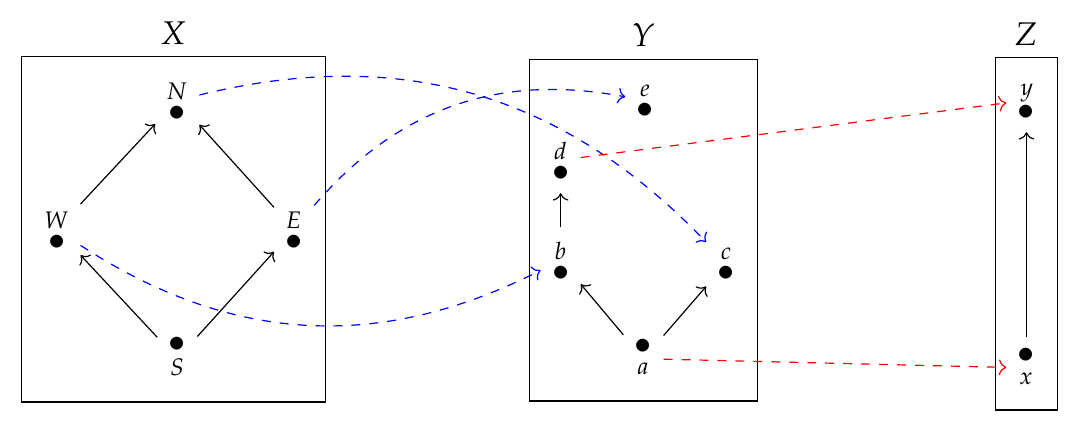In the puzzles [last time](https://forum.azimuthproject.org/discussion/2281/lecture-57-chapter-4-feasibility-relations/p1) we saw something nice: a [feasibility relation](https://forum.azimuthproject.org/discussion/2281/lecture-57-chapter-4-feasibility-relations#latest) between preorders is a generalization of something we learned about a long time ago: a [monotone function](https://forum.azimuthproject.org/discussion/1828/lecture-4-chapter-1-galois-connections/p1) between preorders.

But the really cool part is this: given preorders \$$X\$$ and \$$Y\$$, we can get a feasibility relation \$$\Phi : X \nrightarrow Y\$$ either from a monotone function \$$f : X \to Y\$$ or from a monotone function \$$g: Y \to X\$$. So, feasibility relations put monotone functions going _forwards_ from \$$X\$$ to \$$Y\$$ and those going _backwards_ from \$$Y\$$ to \$$X\$$ into a common framework!

Even better, we saw that one of our favorite themes, namely _adjoints_, is deeply connected to this idea. Let me state this as a theorem:

**Theorem.** Let \$$f : X \to Y \$$ and \$$g: Y \to X\$$ be monotone functions between the preorders \$$X\$$ and \$$Y\$$. Define the feasibility relations \$$\Phi : X \nrightarrow Y\$$ by

$\Phi(x,y) \text{ if and only if } f(x) \le y$

and

$\Psi(x,y) \text{ if and only if } x \le g(y) .$

Then \$$\Phi = \Psi\$$ if and only if \$$f \$$ is the left adjoint of \$$g\$$.

**Proof.** We have \$$\Phi = \Psi\$$ iff

$\Phi(x,y) \text{ if and only if } \Psi(x,y)$

for all \$$x \in X, y \in Y\$$, but by our definitions this is true iff

$f(x) \le y \text{ if and only if } x \le g(y)$

which is true iff \$$f\$$ is the left adjoint of \$$g\$$. \$$\qquad \blacksquare \$$

Ah, if only all proofs were so easy!

Now, to make feasibility relations into a truly satisfactory generalization of monotone functions, we should figure out how to _compose_ them. Luckily this is easy, because we already know how to compose relations from [Lecture 40](https://forum.azimuthproject.org/discussion/2223/lecture-40-chapter-3-relations/p1). So, we should try to prove this:

**Theorem.** Suppose that \$$\Phi : X \nrightarrow Y, \Psi : Y \nrightarrow Z\$$ are feasibility relations between preorders. Then there is a **composite** feasibility relation

$\Psi \Phi : X \nrightarrow Z$

defined as follows:

$(\Psi \Phi)(x,z) = \text{true}$

if and only if for some \$$y \in Y\$$,

$\Phi(x,y) = \text{true} \text{ and } \Psi(y,z) = \text{true}.$

**Puzzle 176.** Prove this! Show that \$$\Psi \Phi\$$ really is a feasibility relation.

I hope you see how reasonable this form of composition is. Think of it in terms of our pictures from last time:Here we have three preorders \$$X,Y,Z\$$, which we can think of as cities with one-way roads. We can also take one-way airplane flights from \$$X\$$ to \$$Y\$$ and from \$$Y\$$ to \$$Z\$$: the flights in blue are a way of drawing a feasibility relation \$$\Phi: X \nrightarrow Y\$$, and the flights in red are a way of drawing \$$\Psi: Y \nrightarrow Z\$$.

**Puzzle 177.** Is \$$(\Psi\Phi)(N,y) = \text{true}\$$?

**Puzzle 178.** Is \$$(\Psi\Phi)(W,y) = \text{true}\$$?

**Puzzle 179.** Is \$$( \Psi\Phi)(E,y) = \text{true}\$$?

**Puzzle 180.** Prove that there is a category \$$\textbf{Feas}\$$ whose objects are preorders and whose morphisms are feasibility relations, composed as above.

**Puzzle 181.** Suppose that \$$\Phi : X \nrightarrow Y, \Psi : Y \nrightarrow Z\$$ are feasibility relations between preorders. Prove that

$(\Psi\Phi)(x,z) = \bigvee_{y \in Y} \Phi(x,y) \wedge \Psi(y,z)$

where \$$\wedge\$$ is the meet in the poset \$$\textbf{Bool}\$$, and \$$\bigvee\$$ is the join. How is this formula related to matrix multiplication?

You may remember that a feasibility relation is a \$$\mathcal{V}\$$-enriched profunctor in the special case where \$$\mathcal{V} = \textbf{Bool}\$$. This formula will be the key to defining composition for more general \$$\mathcal{V}\$$-enriched profunctors. But we need to talk about that more.

**[To read other lectures go here.](http://www.azimuthproject.org/azimuth/show/Applied+Category+Theory#Chapter_4)**